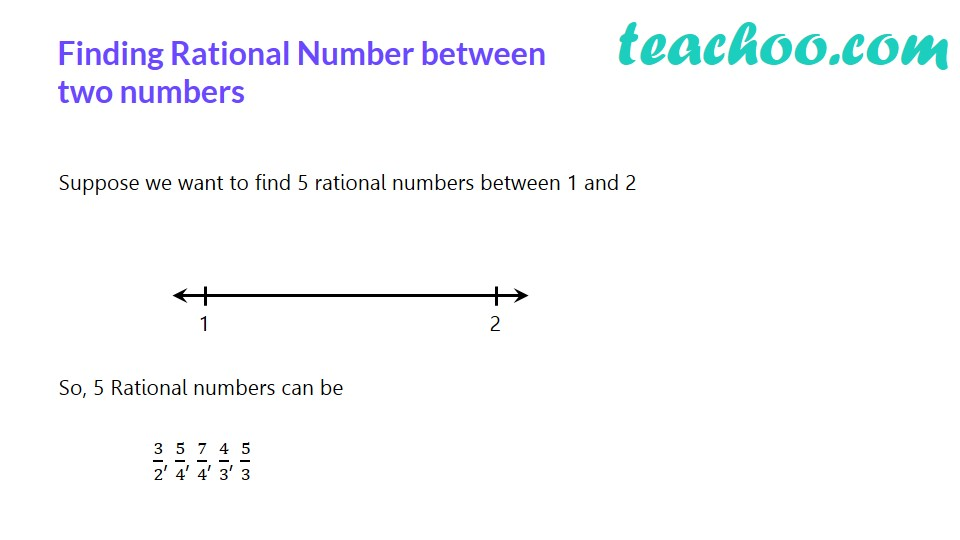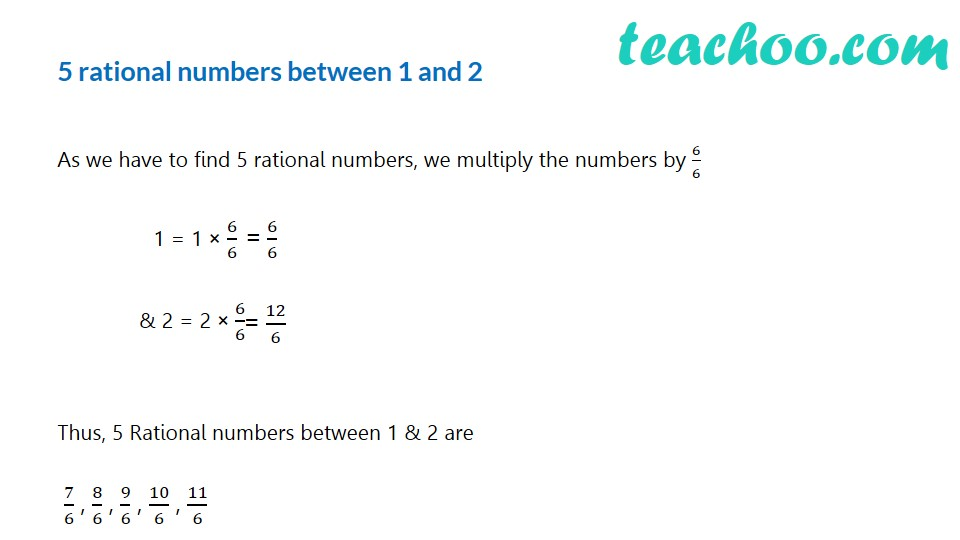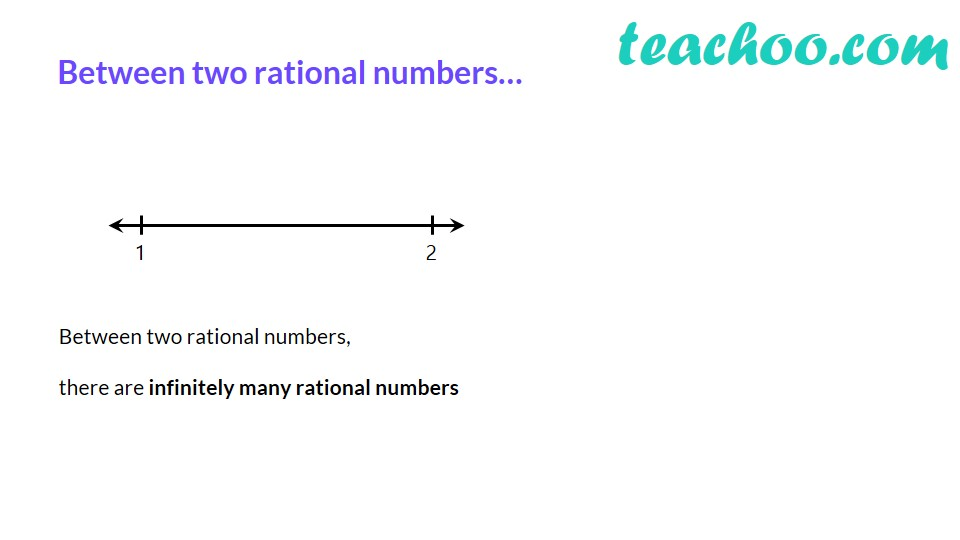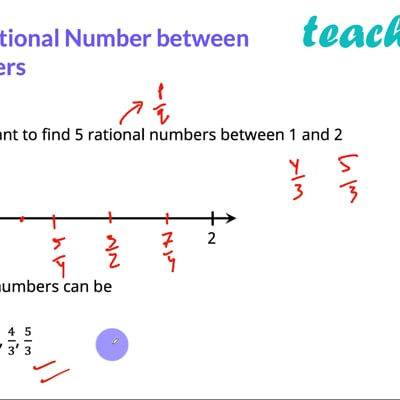Finding rational number between two numbers

Chapter 1 Class 9 Number Systems
Concept wiseThis video is only available for Teachoo black users

Let us take an example on how to do this

## Find five rational numbers between 1 and 2.

As we have to find 5 rational numbers, we multiply the numbers by 6/6

1 = 1 × 6/6 = 6/6

2 = 2 × 6/6 = 12/6

Thus, 5 Rational numbers between 1 & 2 (i.e. 6/6 & 12/6 ) are

7/6, 8/6, 9/6, 10/6, 11/6

Note:

Note that 5/4, 11/8, 13/8, 14/8,……… are also rational numbers between 1 and 2

So, there can be infinitely many rational numbers between any two rational numbers .

Introducing your new favourite teacher - Teachoo Black, at only ₹83 per month

### Transcript

Finding Rational Number between two numbers Suppose we want to find 5 rational numbers between 1 and 2 So, 5 Rational numbers can be 3/2, 5/4, 7/4, 4/3, 5/3 5 rational numbers between 1 and 2 As we have to find 5 rational numbers, we multiply the numbers by 6/6 1 = 1 × 6/6 & 2 = 2 × 6/6 Thus, 5 Rational numbers between 1 & 2 are 7/6 , 8/6 , 9/6 , 10/6 , 11/6